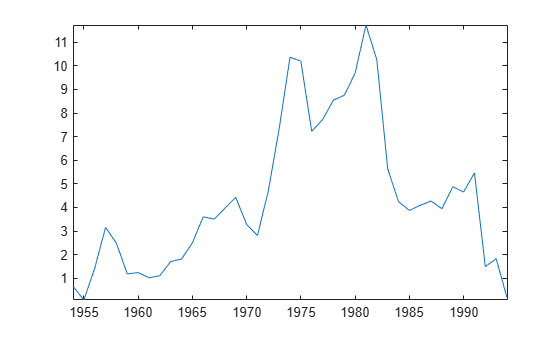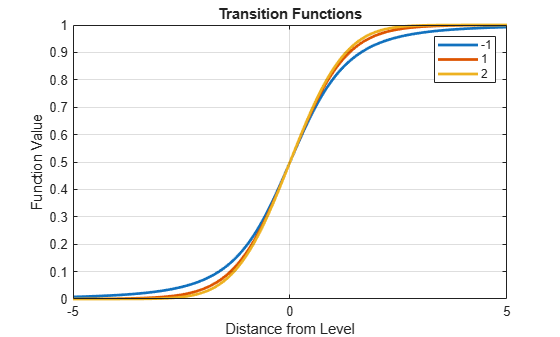# threshold

Create threshold transitions

## Description

`threshold` creates threshold transitions from the specified levels and transition type, either discrete or smooth. Use a `threshold` object to specify the switching mechanism of a threshold-switching dynamic regression model (`tsVAR`).

To study a threshold transitions model, pass a fully specified `threshold` object to an object function. You can specify transition levels and rates as unknown parameters (`NaN` values), which you can estimate when you fit a `tsVAR` model to data by using `estimate`.

Alternatively, to create a random switching mechanism, governed by a discrete-time Markov chain, for a Markov-switching dynamic regression model, see `dtmc` and `msVAR`.

## Creation

### Syntax

``tt = threshold(levels)``
``tt = threshold(levels,Name,Value)``

### Description

example

````tt = threshold(levels)` creates the threshold transitions object `tt` for discrete state transitions specified by the transition mid-levels `levels`.```

example

````tt = threshold(levels,Name,Value)` sets properties using name-value argument syntax. For example, ```threshold([0 1],Type="exponential",Rates=[0.5 1.5])``` specifies smooth, exponential transitions at mid-levels `0` and `1` with rates `0.5` and `1.5`, respectively. ```

### Input Arguments

expand all

Transition mid-levels t1, t2,… tn, specified as an increasing numeric vector.

Levels tj separate threshold variable data into n + 1 states represented by the intervals (−∞,t1),[t1,t2),… [tn,∞).

`NaN` entries indicate estimable transition levels. The `estimate` function of `tsVAR` treats the known elements of `levels` as equality constraints during optimization.

`threshold` stores `levels` in the Levels property

Data Types: `double`

## Properties

expand all

You can set most properties when you create a model by using name-value argument syntax. You can modify only `StateNames` by using dot notation. For example, create a two-state, logistic transition at 0, and then label the first and second states `Depression` and `Recession`, respectively.

```tt = threshold(0,Type="logistic"); tt.StateNames = ["Depression" "Recession"];```

Type of transitions, specified as a character vector or string scalar.

The transition function F(zt,tj,rj) associates a transition type with each threshold level tj, where zt is a threshold variable and rj is a level-specific transition rate. Each function F is bounded between 0 and 1. This table contains the supported types of transitions:

ValueDescription
`"discrete"` (default)

Discrete transitions: $F\left({z}_{t},{t}_{j}\right)=\left\{\begin{array}{ll}0\hfill & ,{z}_{t}<{t}_{j}\hfill \\ 1\hfill & ,{z}_{t}>={t}_{j}\hfill \end{array}.$

Discrete transitions do not have transition rates.

`"normal"`

Cumulative normal transitions: F(zt,tj,rj) = `normcdf(zt,tj,1/rj)`.

`"logistic"`

Logistic transitions: $F\left({z}_{t},{t}_{j},{r}_{j}\right)=\frac{1}{1+{e}^{-{r}_{j}\left({z}_{t}-{t}_{j}\right)}}.$

`"exponential"`

Exponential transitions: $F\left({z}_{t},{t}_{j},{r}_{j}\right)=1-{e}^{-{r}_{j}{\left({z}_{t}-{t}_{j}\right)}^{2}}.$

`"custom"`Custom transition function specified by the function handle of the TransitionFunction property.

Example: `"normal"`

Data Types: `char` | `string`

Transition mid-levels t1, t2,… tn, specified as an increasing numeric vector. The `levels` input argument sets `Levels`.

Data Types: `double`

Transition rates r1, r2,… rn, specified as a positive numeric vector of length n, the number of levels. Each rate corresponds to a level in `Levels`.

`NaN` values indicate estimable rates. The `estimate` function of `tsVAR` treats the known elements of `Rates` as equality constraints during optimization.

`threshold` ignores rates for discrete transitions.

Example: `[0.5 1.5]`

Data Types: `double`

Number of states, specified as a positive scalar and derived from `Levels`.

Data Types: `double`

State labels, specified as a string vector, cell vector of character vectors, or numeric vector of length `numStates`.

`StateNames(1)` names the state corresponding to (−∞,t1), `StateName(2)` names the state corresponding to [t1,t2),… and `StateNames(n + 1)` names the state corresponding to [tn,∞).

Example: ```["Depression" "Recession" "Stagnant" "Boom"]```

Data Types: `string`

Custom transition function F(zt,tj,rj), specified as a function handle. The handle must specify a function with the following syntax:

`function f = transitionfcn(z,tj,rj)`
where:

• You can replace the name `transitionfcn`.

• `z` is a numeric vector of threshold variable data.

• `tj` is a numeric scalar threshold level.

• `rj` is a numeric scalar rate.

• `f` is a numeric vector of in the interval [0,1].

When `Type` is not `"custom"`, `threshold` ignores `TransitionFunction`.

Example: `@transitionfcn`

Data Types: `function_handle`

## Object Functions

 `ttplot` Plot threshold transitions `ttdata` Transition function data `ttstates` Threshold variable data state path

## Examples

collapse all

Create a threshold transition at mid-level ${\mathit{t}}_{1}=0$.

```t1 = 0; tt = threshold(t1)```
```tt = threshold with properties: Type: 'discrete' Levels: 0 Rates: [] StateNames: ["1" "2"] NumStates: 2 ```

`tt` is a `threshold` object representing a discrete threshold transition at mid-level `0`. tt is fully specified because its properties do not contain `NaN` values. Therefore, you can pass `tt` to any `threshold` object function.

Given a univariate threshold variable, `tt` divides the range of the variable into two distinct states, which `tt` labels `"1"` and `"2"`. `tt` also specifies the switching mechanism of a threshold-switching autoregressive (TAR) model, represented by a `tsVAR` object. Given values of the observed univariate transition variable:

• The TAR model is in state `"1"` when the transition variable is in the interval $\left(-\infty ,0\right)$.

• The TAR model is in state `"2"` when the transition variable is in the interval $\left[0,\infty \right)$.

This example shows how to create two logistic threshold transitions with different transition rates, and then display a gradient plot of the transitions.

Load the yearly Canadian inflation and interest rates data set. Extract the inflation rate based on consumer price index (`INF_C`) from the table, and plot the series.

```load Data_Canada INF_C = DataTable.INF_C; plot(dates,INF_C); axis tight```Assume the following characteristics of the inflation rate series:

• Rates below 2% are low.

• Rates at least 2% and below 8% are medium.

• Rates at least 8% are high.

• A logistic transition function describes the transition between states well.

• Transition between low and medium rates are faster than transitions between medium and high.

Create threshold transitions to describe the Canadian inflation rates.

```t = [2 8]; % Thresholds r = [3.5 1.5]; % Transition rates statenames = ["Low" "Med" "High"]; tt = threshold(t,Type="logistic",Rates=r,StateNames=statenames)```
```tt = threshold with properties: Type: 'logistic' Levels: [2 8] Rates: [3.5000 1.5000] StateNames: ["Low" "Med" "High"] NumStates: 3 ```

Plot the threshold transitions; show the gradient of the transition function between the states, and overlay the data.

```figure ttplot(tt,Data=INF_C)```A threshold-switching dynamic regression (`tsVAR`) model has two main components:

• Threshold transitions, which represent the switching mechanism between states. Mid-levels and transition rates are estimable.

• A collection of autoregressive models describing the dynamic system among states. Submodel coefficients and covariances are estimable.

Before you create a threshold-switching model, you must specify its threshold transitions by using `theshold`. If you plan to fit a threshold-switching model to data, you can fully specify its threshold transitions if you know all mid-levels and transition rates. If you need to estimate some or all mid-levels and rates, you can enter `NaN` values as placeholders for unknown parameters. `estimate` treats all specified parameters as equality constraints during estimation. Regardless, to fit threshold transition parameters to data, you must specify a partially specified `threshold` object as the switching mechanism of a threshold-switching model.

Prepare All Estimable Parameters for Estimation

Consider a smooth transition autoregressive (STAR) model that switches between three states (two thresholds) with an exponential transition function.

Create the switching mechanism. Specify that all estimable parameters are unknown.

```t1 = [NaN NaN]; % Two unknown mid-levels r1 = [NaN NaN]; % Two unknown transition rates tt1 = threshold(t1,Type="exponential",Rates=r1)```
```tt1 = threshold with properties: Type: 'exponential' Levels: [NaN NaN] Rates: [NaN NaN] StateNames: ["1" "2" "3"] NumStates: 3 ```

`tt` is a partially specified `threshold` object to pass to `tsVAR` as the switching mechanism. The `estimate` function of `tsVAR` fits the two mid-levels and transition rates to the data with any unknown submodel parameters in the threshold-switching model.

Specify Equality Constraints

Consider a STAR model, which has a switching mechanism with the following qualities:

• The thresholds are at -1 and 1.

• The transition function is exponential.

• The transition rate between the first and second state is 0.5, but the rate between the second and third states is unknown.

Create the switching mechanism.

```t2 = [-1 1]; r2 = [0.5 NaN]; tt2 = threshold(t2,Type="exponential",Rates=r2)```
```tt2 = threshold with properties: Type: 'exponential' Levels: [-1 1] Rates: [0.5000 NaN] StateNames: ["1" "2" "3"] NumStates: 3 ```

`tt` is a partially specified `threshold` object to pass to `tsVAR` as the switching mechanism. The `estimate` function of `tsVAR` does the following:

• Treat the mid-levels `tt.Levels` and the first transition rate `tt.Rates(1)` as equality constraints

• Fit the second transition rate `tt.Rates(2)` to the data with any unknown submodel parameters in the threshold-switching model

Create smooth threshold transitions with the following qualities:

• Mid-levels are at -1, 1, and 2.

• The transition function is the Student's $\mathit{t}$ cdf, which allows for a more gradual mixing than the normal cdf.

• The transition rates, which are the degrees of freedom of the distribution, are 3, 10, and 100.

```t = [-1 1 2]; r = [3 10 100]; ttransfcn = @(z,ti,ri)tcdf(z,ri); tt = threshold(t,Type="custom",TransitionFunction=ttransfcn,Rates=r)```
```tt = threshold with properties: Type: 'custom' Levels: [-1 1 2] Rates: [3 10 100] StateNames: ["1" "2" "3" "4"] NumStates: 4 ```

Plot graphs of each transition function.

```figure ttplot(tt,Type="graph") legend(string(tt.Levels))```• To widen a smooth transition band to show a more gradual mixing of states, decrease the transition rate by specifying the `Rates` name-value argument when you create threshold transitions.